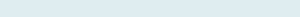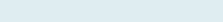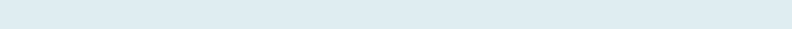# Return Next Cell Value (Min and Max criteria)

Understand how you can find the Text that fulfill Minimum and Maximum criteria from next given column.

In the first Example you will evaluate Minimum example in detail and the we will quickly wrap up the Max example as well.

Query: We have columns, Student and Five School days. Our task is to return the name of Student who got lowest marks each Day.

Formula to implement:

=INDEX(\$B\$3:\$B\$11,MATCH(MIN(C3:C11),C3:C11,0),)

Functions applicable:

In both example we will be creating our formula with Index and Match along with MIN or MAX function in Excel:

• MATCH: Searches for a specified item in a range of cells, and then returns the relative position of that item in the range.
• INDEX: Returns the value at a given position in a range or array. You can use the index to retrieve individual values or entire rows and columns.
• MIN: The Excel MIN function returns the smallest numerical with the lowest value as per given reference of cell range.

Formula Explanation (Step by Step)

Excel start calculating from the innermost parenthesis and then work outward. You can also assess debug formula using shortcut Key F9 function key.

Let’s break the formula into multiple small steps to understand its application.

First formula:

MIN(C3:C11)

Syntax: =MIN (number1, [number2], …)

Step 1:Result: 9.7

Returns minimum value in the given range (C3 to C11)

Second formula:

MATCH(MIN(C3:C11),C3:C11,0),)

Syntax: MATCH(lookup_value, lookup_array, [match_type])

Step 2:Result: 7

It returns the position of lookup value, Cell C9 (9.7) is 7th in the given range (C3 to C11).

Third formula:

INDEX(\$B\$3:\$B\$11,MATCH(MIN(C3:C11),C3:C11,0),)

Syntax: INDEX(array, row_num, [column_num]

Step 3: INDEX(\$B\$3:\$B\$11,7)Result: Sally

Find Name of position Seven in the given range (B3 to B11).

## Find Name that with Maximum Value

All steps are followed as for Minimum example above the only change is Maximum function in the formula.

Formula Applied to Get ‘Maximum’

=INDEX(\$B\$3:\$B\$11,MATCH(MAX(C3:C11),C3:C11,0),)

Result: Sarah      ‘Monday

It first gives the Max (20.5) value in the range (C3 to C11) then Match returns the position (6) of lookup value and now Index will return value (Sarah) of the given position.

• Vlookup cannot look up to its right. Index and Match does not have such problem.
• If you want to look into more Students on top or bottom, try to use filter tool of Excel under Data tab.